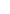## D.C. Circuits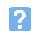1. Which one of the following gives the unknown current in the circuit shown below?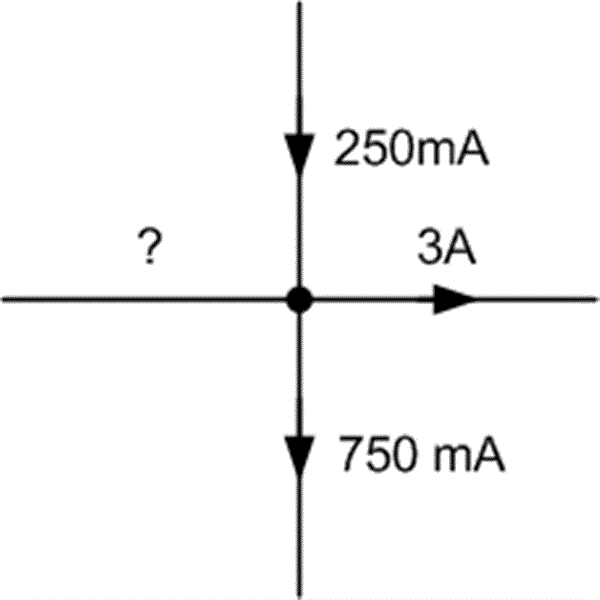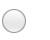a) 2.5A flowing away from the junctionb) 2.75A flowing away from the junctionc) 3.25A flowing towards the junctiond) 3.5A flowing towards the junction.2. Which of the following gives the unknown current in the circuit shown below?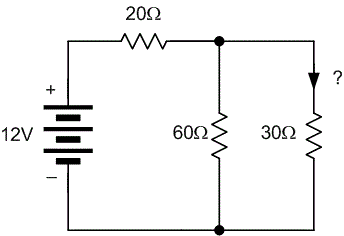a) 10 mAb) 20 mAc) 100 mAd) 200 mA.3. Which one of the following gives the output voltage produced by the circuit shown below?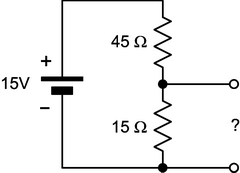a) 3.75 Vb) 5 Vc) 6.25 Vd) 11.25 V.4. A moving coil meter has a full scale deflection current of 1 mA and a coil resistance of 200 ohm. What value of shunt resistance is required to make the meter read 5 mA full-scale?a) 4 ohmb) 25 ohmc) 40 ohmd) 50 ohm.5. The Thevenin equivalent circuit consists of:a) an ideal voltage source connected in series with a resistanceb) an ideal voltage source connected in parallel with a resistancec) an ideal current source connected in series with a resistanced) an ideal current source connected in parallel with a resistance.6. The Norton equivalent circuit consists of:a) an ideal voltage source connected in series with a resistanceb) an ideal voltage source connected in parallel with a resistancec) an ideal current source connected in series with a resistanced) an ideal current source connected in parallel with a resistance.7. The Wheatstone bridge shown below will be balanced when the value of the unknown resistance is: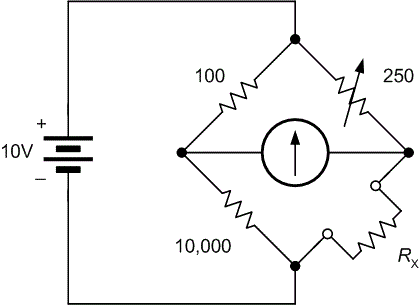a) 2.5 ohmb) 25 ohmc) 2.5 kohmd) 25 kohm.8. A C-R circuit is usually considered to be fully charged after a period of time equal to:a) 0.5 CRb) CRc) 2CRd) 5CR.9. An initially uncharged 5 uF capacitor is charged from a 10 V supply through a resistor of 2 Mohm. After 10 s the voltage across the capacitor will be approximately:a) 3.7 Vb) 5 Vc) 6.3 Vd) 7.5 V.10. A square wave at 100Hz is applied to the input of an C-R integrating circuit which has a time constant of 1s. Which one of the following gives the shape of the output waveform?a) A sine waveb) A pulse wavec) A triangle waved) A much smaller square wave.
This is more feedback!
This is the feedback!